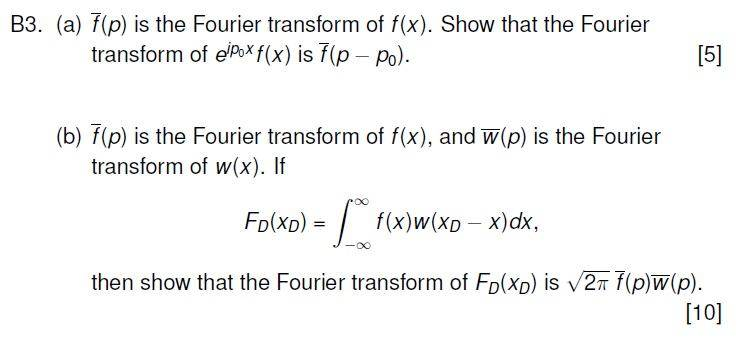# Manipulating Fourier transforms

## Homework Statement## The Attempt at a Solution

##\widetilde{f}(p) = \frac{1}{\sqrt{2\pi}} \int_{-\infty}^{\infty} \! e^{-ipx}f(x) \, \mathrm{d}x##

Then moving on to the transform for ##e^{ip_0 x}f(x)## I get:

##\frac{1}{\sqrt{2\pi}} \int_{-\infty}^{\infty} \! e^{-ipx}e^{ip_0 x}f(x) \, \mathrm{d}x##

## = \frac{1}{\sqrt{2\pi}} \int_{-\infty}^{\infty} \! e^{-i(p-p_0)x}f(x) \, \mathrm{d}x##

## =\widetilde{f}(p-p_0)##

However I'm really not sure what to do for part b).

Here's my attempt so far:

Starting with:

##F_D(x_D) = \int_{-\infty}^{\infty} \! f(x)\Big[w(x_D - x)\Big] \, \mathrm{d}x##

The ##w(x_D - x)## part is similar to the previous section so I plug that in to get:

##F_D(x_D) = \int_{-\infty}^{\infty} \! f(x) \, \Big[\frac{1}{\sqrt{2\pi}} \int_{-\infty}^{\infty} \! e^{-i(x_D -x)p}\widetilde{w}(p) \, \mathrm{d}p\Big] \mathrm{d}x##

then rearrange a little to get:

##F_D(x_D) = \Big(\frac{1}{\sqrt{2\pi}}\int_{-\infty}^{\infty} \! e^{ixp}f(x) \, \mathrm{d}x\Big) \int_{-\infty}^{\infty} \! e^{-ix_D p}\widetilde{w}(p) \, \mathrm{d}p##

The part in brackets is the transform giving ##\widetilde{f}(p)## so,

##F_D(x_D) = \widetilde{f}(p) \int_{-\infty}^{\infty} \! e^{-ix_D p}\widetilde{w}(p) \, \mathrm{d}p##

which can be neatened a little into:

##F_D(x_D) = \widetilde{f}(p)\widetilde{w}(p) \int_{-\infty}^{\infty} \! e^{-ix_D p} \, \mathrm{d}p##

so now I have ##F_D(x_D)## written in a way that's already starting to look like the desired outcome. However it only gets messy now as I do the fourier transform:

##\frac{1}{\sqrt{2\pi}} \int_{-\infty}^{\infty} \! e^{ix_D p}\Big[F_D(x_D)\Big] \, \mathrm{d}x_D = \frac{1}{\sqrt{2\pi}} \int_{-\infty}^{\infty} \! e^{ix_D p}\Big[\widetilde{f}(p)\widetilde{w}(p) \int_{-\infty}^{\infty} \! e^{-ix_D p} \, \mathrm{d}p\,\Big] \mathrm{d}x_D##

The exponents cancel to leave

##\frac{1}{\sqrt{2\pi}} \widetilde{f}(p)\widetilde{w}(p) \int_{-\infty}^{\infty} \! \int_{-\infty}^{\infty} \! \, \mathrm{d}p\, \mathrm{d}x_D##

which I really don't think is a very good result!

Any input would be much appreciated. Thanks.

Last edited:

BvU
Homework Helper
which can be neatened a little into:
Can you explain why it is allowed to move ##\tilde w(p)## in front of the integral sign ?

•sa1988
Can you explain why it is allowed to move ##\tilde w(p)## in front of the integral sign ?

Ah, I see now, it can't be shifted over because it's a function of p so it needs to stay within the integral over dp.

Furthermore I've just been looking through some old lecture notes and it turns out I was very much on the right track (woohoo!). The question is essentially proving the convolution theorem.

So I'd got to the point:

##F_D(x_D) = \widetilde{f}(p)\int_{-\infty}^{\infty} \! e^{-ix_D p}\widetilde{w}(p) \, \mathrm{d}p##

which actually neatens up to

##F_D(x_D) = \int_{-\infty}^{\infty} \! e^{-ix_D p}\widetilde{f}(p)\widetilde{w}(p) \, \mathrm{d}p##

which can be made into a standard fourier transform by adding factors of ##\sqrt{2\pi}##:

##\frac{1}{\sqrt{2\pi}}F_D(x_D) = \frac{1}{\sqrt{2\pi}}\int_{-\infty}^{\infty} \! e^{-ix_D p}\widetilde{f}(p)\widetilde{w}(p) \, \mathrm{d}p##

then taking the inverse:

##\frac{1}{\sqrt{2\pi}}\widetilde{F}_D(p) = \widetilde{f}(p)\widetilde{w}(p)##

which clearly leads to the desired result:

##\widetilde{F}_D(p) = \sqrt{2\pi}\widetilde{f}(p)\widetilde{w}(p)##